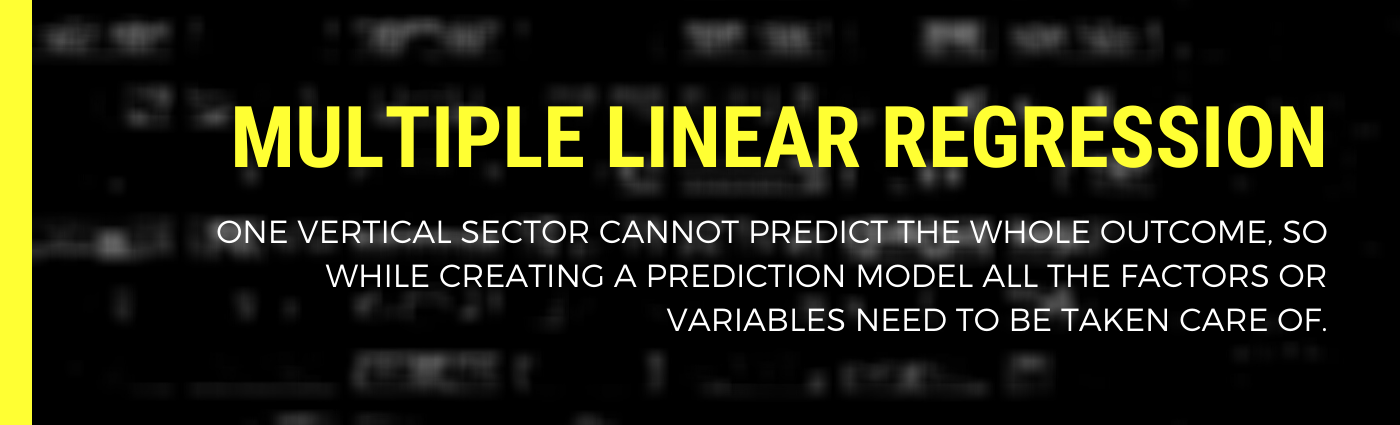# Multiple Linear Regression

Original article was published by Nahid Fatma on Artificial Intelligence on MediumIn the previous article, we talked about Simple Linear Regression where we were able to do prediction by using one variable only but as we advance to the field of Machine Learning, we realize that real-world outcomes does not depend only one a single variable but tend to stretch across a lot. When the relation between the variables is linear and we have more than one independent variable affecting the dependent variable, then multiple regression is used for creating the predictive model.

Now to quote it analytically, Multiple Linear Regression is a statistical technique that uses several explanatory (independent) variables to predict the outcome of a response (dependent) variable. The mathematical formula for this statistical technique is given below.

Y = a + b1X1 + b2X2 + ……… + bnXn

Y: dependable variable

X1, X2, ….., Xn: independent variable

a: random error

b1, b2, …… , bn : regression coefficient

All the statistical techniques are developed considering few assumptions, the two most important assumption involved with Multiple Linear Regression are:

1. There is a linear relationship between the dependent and independent variables.
2. The correlation between the dependent variables is very low.

Since we are done with learning the concepts, let’s do some hands-on Multiple linear regression in python.

Step 1: Import the data set

Here I have used the pandas library for reading my CSV file. X is assigned with the data frame containing all the independent variable or factors.

import pandas as pd

X = dataset.iloc[:, :-1].values
y = dataset.iloc[:, 4].values

Step 2: Encode the categorical data, if any.

Data are of two main types: Categorical and Numerical which can be further segmented into Nominal and ordinal for categorical and discrete and continuous for Numerical data. More information about the forms of these data is in figure 2 and figure 3.

Machine Learning models require input in numeric form but when we have categorical data in our data set we convert it into numeric data which is called encoding. Here I am using one-hot encoding technique for my categorical data, for that we can use LabelEncoder and OneHotEncoder from sklearn library.

from sklearn.preprocessing import LabelEncoder, OneHotEncoder
labelencoder = LabelEncoder()
X[:, 3] = labelencoder.fit_transform(X[:, 3])
onehotencoder = OneHotEncoder(categorical_features = )
X = onehotencoder.fit_transform(X).toarray()

As you all can see in the code, I am removing one row from the dataframe to avoid a dummy variable trap which is a scenario that happens when two or more variables get highly correlated. (one variable can be predicted with others) So the solution of this trap is to always drop one of the categorical variables after encoding.

Step 3: Splitting the dataset into the Training set and Test set

from sklearn.model_selection import train_test_split
X_train, X_test, y_train, y_test = train_test_split(X, y, test_size = 0.2, random_state = 0)

Step 4: Fitting Multiple Linear Regression to the Training set.

from sklearn.linear_model import LinearRegression
regressor = LinearRegression()
regressor.fit(X_train, y_train)

Step 5: Predicting the test set results

y_pred = regressor.predict(X_test)

So, in just five simple steps we can build a Multiple Linear Regression Model. We can save this code as a template and can use it for writing regression models with different datasets.